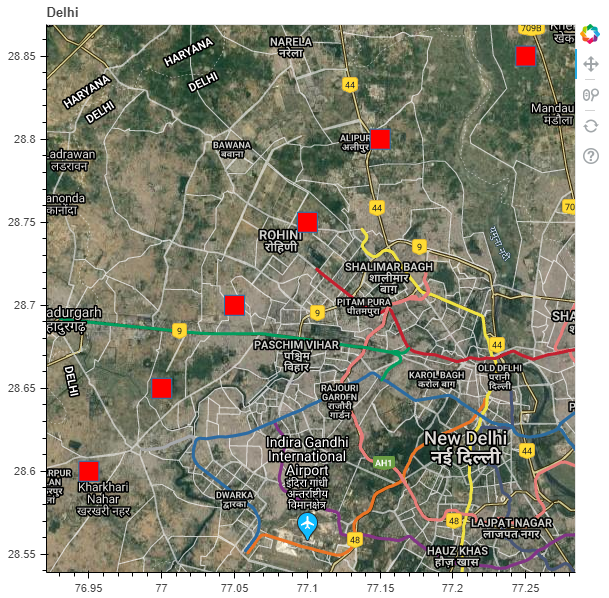# Python Bokeh – Plotting glyphs over a Google Map

Bokeh is a Python interactive data visualization. It renders its plots using HTML and JavaScript. It targets modern web browsers for presentation providing elegant, concise construction of novel graphics with high-performance interactivity.

Bokeh can be used to plot glyphs over a Google map. A glyph is a hieroglyphic character or a symbol. To use Google maps in Bokeh, we will use the `gmap()` function of the `plotting` class.

## gmap()

Syntax : gmap(parameters)
Parameters :

• map_options : GMapOptions i.e. the configuration specific to the Google map
• active_drag : drag tool that is to be initially active
• active_inspect : inspect tool that is to be initially active
• active_scroll : scroll tool that is to be initially active
• active_tap : tap tool that is to be initially active
• title : title of the Google map
• x_axis_label : label for the x-axis
• x_axis_location : location of the x-axis
• x_minor_ticks : number of minor ticks between adjacent x-axis major ticks
• y_axis_label : label for the y-axis
• y_axis_location : location of the y-axis
• y_minor_ticks : number of minor ticks between adjacent y-axis major ticks

Returns : an object of the class GMap

Now let us see how to configure the Google map using the `GMapOptions()` function :

## GMapOptions()

Syntax : GMapOptions(parameters)
Parameters :

• lat : latitude where the Google map is to be centered
• lng : longitude where the Google map is to be centered
• map_type : type of map to be used for the plot, here are the map types available :
• hybrid
• satellite
• terrain
• name : name for the Google map
• scale_control : whether the Google map should display its distance scale control
• styles : style of map to be used for the plot
• subscribed_events : list of events that are subscribed to by Python callbacks
• tags : tags associated with the Google map model
• tilt : tilting the angle of the map
• zoom : initial zoom level of the Google map
• apply_theme : theme of the model

Returns : an object of the class GMapOptions

## Let us see how to plot glyphs over a Google Map :

1. Import the required libraries and modules :
• gmap from bokeh.plotting
• ColumnDataSource and GMapOptions from bokeh.models
• output_file and show from bokeh.io
2. Create a file to store our model using `output_file()`.
3. Configure the Google map using `GMapOptions()`.
4. Generate a GoogleMap object using `gmap()`.
5. Determine the coordinates of the glyphs using `ColumnDataSource()`.
6. Generate the glyphs on the created Google map object.
7. Display the Google map using `show()`.

 `# importing the required modules ` `from` `bokeh.plotting ``import` `gmap ` `from` `bokeh.models ``import` `ColumnDataSource, GMapOptions ` `from` `bokeh.io ``import` `output_file, show ` ` `  `# file to save the model ` `output_file(``"gfg.html"``) ` ` `  `# configuring the Google map ` `lat ``=` `28.7041` `lng ``=` `77.1025` `map_type ``=` `"hybrid"` `zoom ``=` `11` `google_map_options ``=` `GMapOptions(lat ``=` `lat, ` `                                 ``lng ``=` `lng, ` `                                 ``map_type ``=` `map_type, ` `                                 ``zoom ``=` `zoom) ` ` `  `# generating the Google map ` `google_api_key ``=` `"" ` `title ``=` `"Delhi"` `google_map ``=` `gmap(google_api_key, ` `                  ``google_map_options, ` `                  ``title ``=` `title) ` ` `  `# the coordinates of the glyphs ` `source ``=` `ColumnDataSource( ` `    ``data ``=` `dict``(lat ``=` `[``28.6``, ``28.65``, ``28.7``, ``28.75``, ``28.8``, ``28.85``], ` `                ``lon ``=` `[``76.95``, ``77``, ``77.05``, ``77.1``, ``77.15``, ``77.25``])) ` ` `  `# generating the glyphs on the Google map ` `x ``=` `"lon"` `y ``=` `"lat"` `size ``=` `20` `fill_color ``=` `"red"` `fill_alpha ``=` `1` `google_map.square(x ``=` `x, ` `                  ``y ``=` `y, ` `                  ``size ``=` `size, ` `                  ``fill_color ``=` `fill_color, ` `                  ``fill_alpha ``=` `fill_alpha, ` `                  ``source ``=` `source) ` ` `  `# displaying the model ` `show(google_map) `

Output :My Personal Notes arrow_drop_upCheck out this Author's contributed articles.

If you like GeeksforGeeks and would like to contribute, you can also write an article using contribute.geeksforgeeks.org or mail your article to contribute@geeksforgeeks.org. See your article appearing on the GeeksforGeeks main page and help other Geeks.

Please Improve this article if you find anything incorrect by clicking on the "Improve Article" button below.

Article Tags :

Be the First to upvote.

Please write to us at contribute@geeksforgeeks.org to report any issue with the above content.